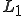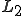# Mutual Inductance of two coaxial coils

## Homework Statement

Two coils of the same length and almost the same cross-section are put one inside the other.
Find their mutual inductance if the self-inductances areand.

## Homework Equations

1) $\phi_i=L_i I_i$
2) $\phi=\iint_A \vec{B} \cdot d\vec{A}$
3) $\phi_1=M_{12} I_2$

## The Attempt at a Solution

[/B]
I've tried working with the equations, substituting one into the other in order to get something that looks like equation 3) but I can't seem to get it...
Thanks,

R

scottdave
Homework Helper
Last edited:
Are those the equations that you have to start from? You may find this useful. http://www.learningaboutelectronics.com/Articles/Mutual-inductance-calculator.php

After reading that, how do you think the fluxes are coupled between the coils in your situation?

One more thing - your title mentions "coaxial" coils, but it doesn't seem that is really what you are asking about.

If one coil is put inside the other, and have the same cross section, are they not coaxial?! Meaning, having the same axis?

scottdave
Homework Helper
If one coil is put inside the other, and have the same cross section, are they not coaxial?! Meaning, having the same axis?
OK. I see your use of the terminology. It made me think of coax style cable, though.

OK. I see your use of the terminology. It made me think of coax style cable, though.
Thanks for the response.
I did read the link you sent me but I can't see how I can use that information.
All i am given are the self inductances of the coils. So i know how to relate between the current flowing through one and the going through it (the same coil).
I tried writing the flux of coil 1 as something that is proportional to the current in coil 2 (and so the proportionality constant will be the mutual inductance). but i know I'm doing something wrong, because everything cancels out and I'm left with $\Phi_1 = L_2 I_1$, which, im pretty sure is not the right answer.

haruspex
Homework Helper
Gold Member
2020 Award
•scottdave
A useful-looking equation is given at that link. All you need is a value for the coupling coeffcient, no?
No.
Firstly, from what i can tell, the geometry of the problem is very different. in the problem i posted, the coils are one inside the other. in the link, they are next to each other.
Secondly, there are no numerical values in my question. what i understand from the question is that i have to find and expression for the mutual inductance using L1 and L2 only.
The relevant equation i wrote down might be in insufficient, and maybe i'm missing something..

Funny how I referenced the same MIT course doc at about the same time?
Thanks, that definitely helps.

haruspex